# Analog and Digital Modulation

## Overview

This tutorial is part of the National Instruments Measurement Fundamentals series. Each tutorial in this series, will teach you a specific topic of common measurement applications, by explaining the theory and giving practical examples. This tutorial covers an introduction to analog and digital modulation.

For the complete list of tutorials, return to the NI Measurement Fundamentals Main page or for more RF tutorials refer to the NI RF Fundamentals Main subpage.

## Modulation Basics

Modulation is a process in which a modulator changes some attribute of a higher frequency carrier signal proportional to a lower frequency message signal. If the carrier is represented by the equation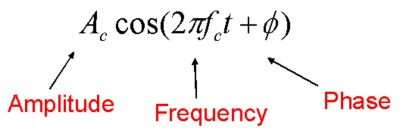Figure 1. Carrier Signal Equation

a change in the message signal will produce a corresponding change in either the amplitude, frequency, or phase of the carrier. A transmitter can then send this carrier signal through the communication medium more efficiently than the message signal alone. Finally, a receiver will demodulate the signal, recovering the original message.

In Amplitude Modulation (AM), pictured below, the amplitude of the carrier sinusoid changes based on the amplitude of the message.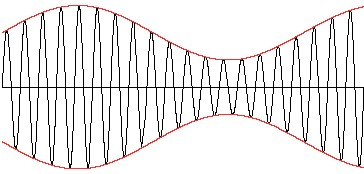Figure 2. Amplitude Modulation

The message signal (red) rides on top of the carrier as the amplitudes of both vary with time. The frequency of the carrier, however, is much higher than the frequency of the message. This carrier frequency is the center of the 'channel,' or frequency allocation of this RF signal. Frequency allocations vary depending on the medium of transmission. For broadcast transmissions, where signals are sent through the air, the government regulates frequency allocation. If the RF signal is transmitted over wire, such as in cable television, there is more freedom in the choice of carrier.

In addition to amplitude modulation, frequency modulation varies the frequency of the carrier sinusoid based on the amplitude of the message signal. Similarly, phase modulation changes the phase of the carrier in response to a change in amplitude of the message.

## Digital Modulation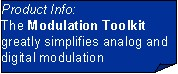Digital modulation is similar to analog modulation, but rather than being able to continuously change the amplitude, frequency, or phase of the carrier, there are only discrete values of these attributes that correspond to digital codes. There are several common digital modulation schemes, each varying separate sets of parameters. The simplest type is called On Off Keying (OOK) where the amplitude of the carrier corresponds to one of two digital states. A nonzero amplitude represents a digital one while a zero amplitude is a digital zero. A specific implementation of OOK is Morse Code. Frequency Shift Keying (FSK), seen in Figure 3, is a form of frequency modulation where a certain frequency represents each binary value.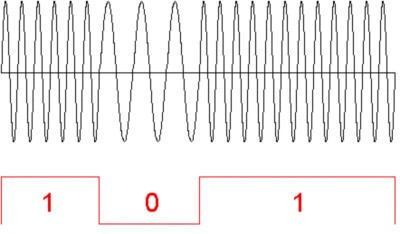Figure 3. Frequency Shift Keying (FSK)

Finally, Quadrature Amplitude Modulation (QAM) uses combinations of amplitudes and phases to represent more than 2 digital states, as many as 1024.

## I and Q Data

Before comparing IQ and IF modulators, review the tutorial: "What is I/Q Data?".

## IQ vs. IF Modulators

After calculating digital I and Q data from the baseband message signal, there are two methods of converting this data into an analog RF signal. The first method involves converting I and Q data into analog signals, then feeding them into a quadrature encoder. There, they control the amplitudes of two oscillators, operating 90 degrees out of phase. The output of these oscillators is summed, resulting in an RF signal with the appropriate amplitude, phase, and frequency.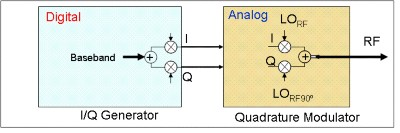Figure 4. IQ Modulation

The next method of converting digital I and Q data to analog RF performs the oscillator scaling and summing in the digital domain. That is, digital sinusoids with a phase difference of 90 degrees are scaled by the digital I and Q values, then added together. These digital sinusoids are of a lower frequency than the analog oscillators in the IQ modulation scheme, but still at a significantly higher frequency than the message signal. A digital to analog converter (DAC), which operates at a much higher frequency than the DAC used in IQ modulation, converts the resulting digital waveform to low frequency analog RF. Finally, an analog IF to RF upconverter uses several stages of mixing and filtering to shift the analog RF signal to the desired RF frequency.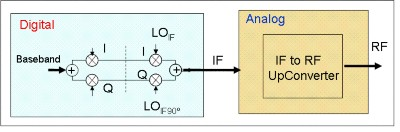Figure 5. IF Modulation

The NI 5660 RF Vectors Signal Analyzer and the NI 5671 RF Vector Signal Generator use the IF modulation scheme depicted in Figure 5.

## Relevant NI Hardware

Customers interested in this topic were also interested in the following NI products:

## Conclusions

This document is meant to provide a brief introduction to analog and digital modulation.

For the complete list of tutorials, return to the NI Measurement Fundamentals Main page or for more RF tutorials refer to the NI RF Fundamentals Main subpage.